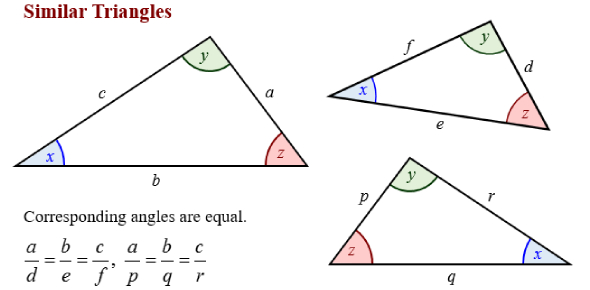# Quiz Similar Triangles

5 Questions | Total Attempts: 1336SettingsIn this mathematical quiz on similar triangles and proportional reasoning, we’ll be offering up proportionally identical triangles and giving you the task of finding “x”, which can be any given side of a triangle. Good luck!

• 1.
Solve for x.
• 2.
Solve for x
• 3.
Solve for x
• 4.
Solve for x
• 5.
A scale model of a building has a scale factor of 1 inch to 25 fee. (On the scale model, each inch represents 25 feet). If the real building has a doorway that is 15 feet tall, how many inches is it on the scale model?
• A.

10 inches

• B.

3/5 inches

• C.

5/3 inches

• D.

375 inches

• E.

Not enough information

Related Topics